Custom SearchCHAPTER 4 LIMITS AND DIFFERENTIATION LEARNING OBJECTIVES Upon completion of this chapter, you should be able to do the following: 1. Define a limit, find the limit of indeterminate forms, and apply limit formulas. 2. Define an infinitesimal, determine the sum and product of infinitesimals, and restate the concept of infinitesimals. 3. Identify discontinuities in a function. 4. Relate increments to differentiation, apply the general formula for differentiation, and find the derivative of a function using the general formula. INTRODUCTION Limits and differentiation are the beginning of the study of calculus, which is an important and powerful method of computation. LIMIT CONCEPT The study of the limit concept is very important, for it is the very heart of the theory and operation of calculus. We will include in this section the definition of limit, some of the indeterminate forms of limits, and some limit formulas, along with example problems. DEFINITION OF LIMIT Before we start differentiation, we must understand certain concepts. One of these concepts deals with the limit of a function. Many times you will need to find the value of the limit of a function. The discussion of limits will begin with an intuitive point of view. We will work with the equation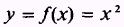which is shown in figure 4-1. Point P represents the point corresponding to y= 16 and x=4 The behavior of y for given values of x near the point x=4 is the center of the discussion. For the present we will exclude point P, which is encircled on the graph. We will start with values lying between and including x=2 and x=6 indicated by interval AB in figure 4-1, view A. This interval may be written as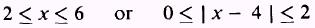The corresponding interval for y is between and includes y=4 and y=36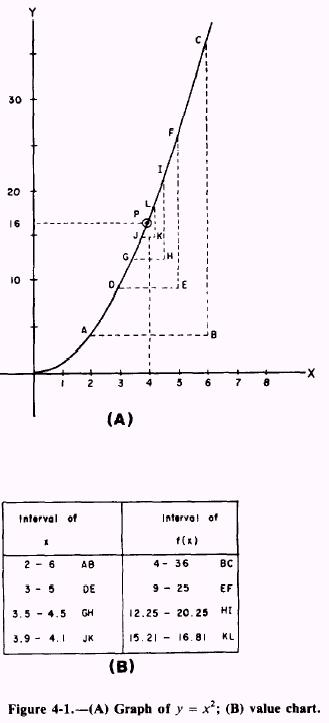We now take a smaller interval, DE, about x = 4 by using values of x=3 and x=5 and find the corresponding interval for y to be between y=9 and y=25 inclusively. These intervals for x and y are written as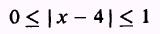andAs we diminish the interval of x around x = 4 (intervals GH and JK) we find the values ofto be grouped more and more closely around y=16 This is shown by the chart in figure 4-1, view B. Although we have used only a few intervals of x in the discussion, you should easily see that we can make the values about y group as closely as we desire by merely limiting the values assigned to x about Because the foregoing is true, we may now say that the limit of x2, as x approaches 4, results in the value 16 for y, and we write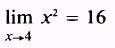In the general form we may write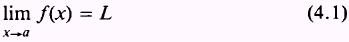Equation (4.1) means that as x approaches a, the limit of f(x) will approach L, where L is the limit of f(x) as x approaches a. No statement is made about f(a), for it may or may not exist, although the limit of f(x), as x approaches a, is defined. We are now ready to define a limit. Let f(x) be defined for all x in the interval near x=a but not necessarily at x=a Then there exists a number, L, such that for every positive number(epsilon), however small,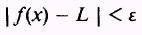provided that we may find a positive number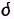(delta) such thatThen we say L is the limit off (x) as x approaches a, and we write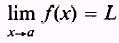This means that for every given number> 0, we must find a numbersuch that the difference between f(x) and L is smaller than the numberwhenever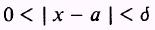EXAMPLE: Suppose we are given= 0.1 andfind a> 0. SOLUTION: We must find a numbersuch that for all points except we have the difference between f(x) and 1 smaller than 0.1. We writeandand we consider only values where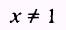Simplifying the first term, we have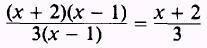Finally, combine terms as follows:so thator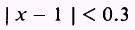Therefore,= 0.3 and we have fulfilled the definition of the limit. If the limit of a function exists, thenSo we can often evaluate the limit by substitution. For instance, to find the limit of the function x2 - 3x + 2 as x approaches 3, we substitute 3 for x in the function. Then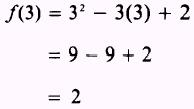Since x is a variable, it may assume a value as close to 3 as we wish; and the closer we choose the value of x to 3, the closer f(x) will approach the value of 2. Therefore, 2 is called the limit of f(x) as x approaches 3, and we write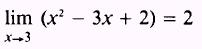Integrated Publishing, Inc. - A (SDVOSB) Service Disabled Veteran Owned Small Business